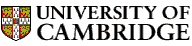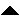Computer LaboratoryComputer Science Syllabus - Discrete Mathematics II (50% option only)Discrete Mathematics II (50% option only)Next: Programming in Java Up: Lent Term 2006: Part Previous: Lent Term 2006: Part   Contents

## Discrete Mathematics II (50% option only)

Lecturer: Professor G.W. Winskel

No. of lectures and mini-seminars: 12 + 4

Prerequisite course: Discrete Mathematics I

This course is a prerequisite for all theory courses as well as Security (Part IB and Part II), Artificial Intelligence I and II, Information Theory and Coding (Part II).

Aims

This part of the course will develop the theory of sets and their uses in Computer Science. The Lent Term half of the Discrete Mathematics course will include a series of mini-seminars involving the active participation of students.

Lectures

• Sets and constructions on sets. Basic sets, comprehension, indexed sets, unions, intersections, products, disjoint unions, powersets. Russell's paradox.

• Relations, functions and partial functions. Composition and identity relations. Lambda notation for functions. Direct and inverse image of a relation. Equivalence relations and partitions. Cardinality especially countability, Cantor's diagonal argument.

• Sets and logic. Powersets as boolean algebras. Characteristic functions. Venn diagrams.

• Well-founded induction. Well-founded relations and well-founded induction. Other induction principles as instances of well-founded induction. Applications.

• Introduction to inductive definitions. Using rules to define sets. Reasoning principles: the inductively define set is the least set closed under the rules; induction on derivations. Simple applications.

Objectives

On completing this part of the course, students should be able to

• understand and use the language of set theory; prove and disprove assertions using a variety of techniques

• understand boolean operations as operations on sets and formulate statements using boolean logic

• apply the principle of well-founded induction

• define sets inductively using rules, and prove properties about them

Comprehensive notes will be provided.

Devlin, K. (2003). Sets, functions, and logic: an introduction to abstract mathematics. Chapman and Hall/CRC Mathematics (3rd ed.).
Biggs, N.L. (1989). Discrete mathematics. Oxford University Press.
Mattson, H.F. Jr (1993). Discrete mathematics. Wiley.
Nissanke, N. (1999). Introductory logic and sets for computer scientists. Addison-Wesley.
Pólya, G. (1980). How to solve it. Penguin.Next: Programming in Java Up: Lent Term 2006: Part Previous: Lent Term 2006: Part   Contents
Christine Northeast
Sun Sep 11 15:46:50 BST 2005© 2005 University of Cambridge Computer Laboratory Please send any comments to pagemaster@cl.cam.ac.uk Page last updated on 11-Sep-2005 at 15:57 by Christine Northeast